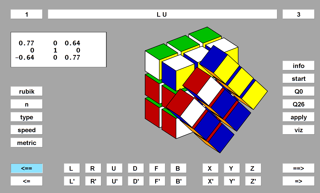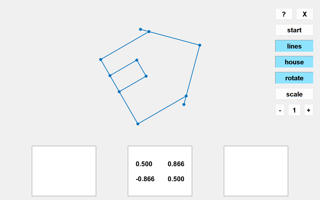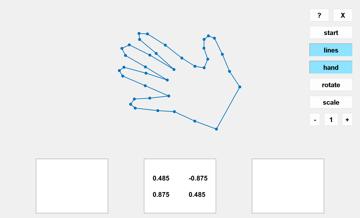# Exploring Matrices Exercises

Try you hand at a few exercises involving Exploring Matrices.

### Contents

#### Qube SimplifiedI have simplified the Qube app by removing these four buttons.

• solve. The <== key now controls the unscrambling operation.
• scramble. The ==> key now does six random rotations.
• order. I never found a satisfactory reference for the group theory of Rubik's cube.
• score. I never found a use for the nuclear norm.

Code for Qube dated 9/24/2023 is included in the Apps mzip archive.

#### Exploring Matrices Exercises

Here are a few exercises for Exploring Matrices. The answers are available at ExMatAnswers.

#### Matrix Multiplication

1. Compute by rows, and by columns.

$$\left( \begin{array}{rrr} 8 & 1 & 6 \\ 3 & 5 & 7 \\ 4 & 9 & 2 \end{array} \right) \left( \begin{array}{r} 1 \\ 1 \\ 1 \end{array} \right)$$

2. Solve for $z$ using inner products of rows, and using linear combinations of columns.

$$\left( \begin{array}{rrr} 1 & 2 & 3 \\ 4 & 5 & 6 \\ 7 & 8 & 9 \end{array} \right) \left( \begin{array}{r} 1 \\ z \\ 1 \end{array} \right) \ = \ \left( \begin{array}{r} 0 \\ 0 \\ 0 \end{array} \right)$$

3. What do the m, n and p buttons on the Multiply app do? What are the other buttons and what do they do?

4. If A is n-by-n and x is n-by-1, how many multiplications are required to compute A x ?

5. If A is m-by-n and B is n-by-p, how many multiplications are required to compute A B ?

#### Rotations and Scaling

1. What is R(30º)?

$$R(\theta) \ = \ \left( \begin{array}{rr} \cos{\theta} & \sin{\theta} \\ -\sin{\theta} & \cos{\theta} \end{array} \right)$$

2. Explain https://xkcd.com/184.

3. What is the value of $\theta$ ?

$$R(\theta) \ = \ \left( \begin{array}{rr} 0.8 & 0.6 \\ -0.6 & 0.8 \end{array} \right)$$

4. What is the value of $\theta$ ?5. Edit a copy of Rotate.m and replace the house with a hand. You can use my hand or your own hand; see exercise 3.4 in Numerical Computing with MATLAB .#### Computer Graphics

1. Show how homogeneous coordinates and matrix-vector multiplication by Tx, Ty or Tz produce translation.

2. What is the range of the rotations used by the pitch, roll, and yaw buttons on the Grafix app?

3. What color in the beacon on top of the plane? How would you change the beacon's color?

4, What is the function of the resolution and offset sliders for the teapot?

5, How many times does the bucky ball bounce off the sides of the plot window?

#### Matrices and Cubes

1. What is the color of central cubelet in the Color Cube?

2. What do the "<=" , "<==" , "=>" and "==>" buttons on Qube do?

3. What is "God's Number" for a 3-by-3-by-3 Rubik's Cube? What are Q20 and Q26? See Cleve's Corner 2022/09/05.

4. Can you restore the following scrambled cubes with fewer moves than <==, the unscramble key? Use the quarter-turn metric and reset the cube with start or by clicking on stack and Q0. You might also want to set speed to 30 or 45,

• LRUDFB
• LRL'R'
• FLLLRB
• Q26
• Reset the random number generator by entering rng(r) for some small integer r in the command window and then generate six random rotations with the ==> key.

Published with MATLAB® R2023a

|

### 댓글

댓글을 남기려면 링크 를 클릭하여 MathWorks 계정에 로그인하거나 계정을 새로 만드십시오.